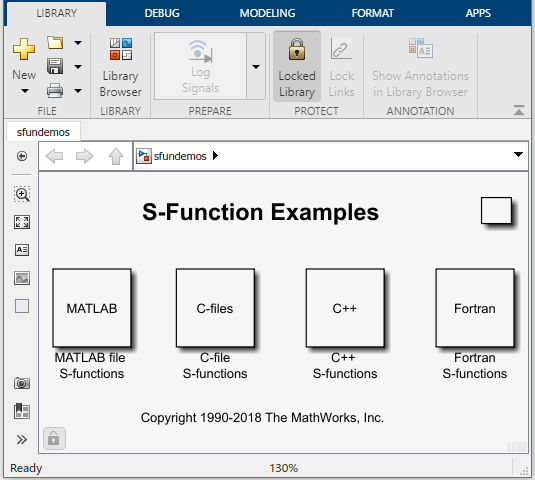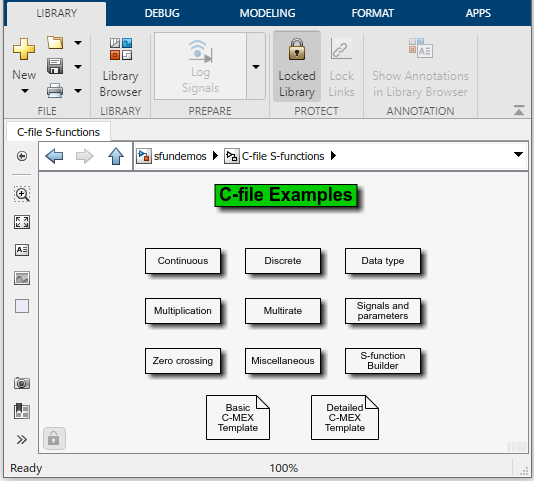Documentation

## S-Function Examples

### Overview of Examples

To run an example:

1. In the MATLAB® Command Window, enter `sfundemos`.

The S-function example library opens.Each block represents a category of S-function examples.

2. Double-click a category to display the examples that it includes. For example, click .3. Double-click a block to open and run the example that it represents.

It might be helpful to examine some sample S-functions as you read the next chapters. Code for the examples is stored in the following folder under the MATLAB root folder.

 MATLAB code `toolbox/simulink/simdemos/simfeatures` C, C++, and Fortran code `toolbox/simulink/simdemos/simfeatures/src`

### Level-2 MATLAB S-Function Examples

The `matlabroot/toolbox/simulink/simdemos/simfeatures` folder (open) contains many Level-2 MATLAB S-functions. Consider starting off by looking at these files.

FilenameModel NameDescription
`msfcn_dsc.m``msfcndemo_sfundsc1`Implement an S-function with an inherited sample time.
`msfcn_limintm.m``msfcndemo_limintm`Implement a continuous limited integrator where the output is bounded by lower and upper bounds and includes initial conditions.
`msfcn_multirate.m``msfcndemo_multirate`Implement a multirate system.
`msfcn_times_two.m``msfcndemo_timestwo`Implement an S-function that doubles its input.
`msfcn_unit_delay.m``msfcndemo_sfundsc2`Implement a unit delay.
`msfcn_varpulse.m``msfcndemo_varpulse`Implement a variable pulse width generator by calling `set_param` from within a Level-2 MATLAB S-function. Also demonstrates how to use custom set and get methods for the block SimState.
`msfcn_vs.m``msfcndemo_vsfunc`Implement a variable sample time block in which the first input is delayed by an amount of time determined by the second input.

### Level-1 MATLAB S-Function Examples

The `matlabroot/toolbox/simulink/simdemos/simfeatures` folder (open) also contains many Level-1 MATLAB S-functions, provided as reference for legacy models. Most of these Level-1 MATLAB S-functions do not have associated example models.

FilenameDescription
`csfunc.m`Define a continuous system in state-space format.
`dsfunc.m`Define a discrete system in state-space format.
`limintm.m`Implement a continuous limited integrator where the output is bounded by lower and upper bounds and includes initial conditions.
`mixedm.m`Implement a hybrid system consisting of a continuous integrator in series with a unit delay.
`sfun_varargm.m`Implement an S-function that shows how to use the MATLAB command `varargin`.
`vsfunc.m`Illustrate how to create a variable sample time block. This S-function implements a variable step delay in which the first input is delayed by an amount of time determined by the second input.

### C S-Function Examples

The `matlabroot/toolbox/simulink/simdemos/simfeatures/src` folder (open) contains examples of C MEX S-functions, many of which have a MATLAB S-function counterpart. The C MEX S-functions are listed in the following table.

FilenameModel NameDescription
`csfunc.c``sfcndemo_csfunc`Implement a continuous system.
`dlimintc.c`No model availableImplement a discrete-time limited integrator.
`dsfunc.c``sfcndemo_dsfunc`Implement a discrete system.
`limintc.c`No model availableImplement a limited integrator.
`mixedm.c``sfcndemo_mixedm`Implement a hybrid dynamic system consisting of a continuous integrator (1/s) in series with a unit delay (1/z).
`mixedmex.c``sfcndemo_mixedmex`Implement a hybrid dynamic system with a single output and two inputs.
`slexQuantizeSFcn.c``sfcndemo_sfun_quantize`Implement a vectorized quantizer. Quantizes the input into steps as specified by the quantization interval parameter, `q`.
`sdotproduct.c``sfcndemo_sdotproduct`Compute dot product (multiply-accumulate) of two real or complex vectors.
`sfbuilder_bususage.c``sfbuilder_bususage`Access S-Function Builder with a bus input and output.
`sfbuilder_movingAverage.c``sfbuilder_movingAverage`Implement simple time window moving average using Start and Terminate.
`sftable2.c`No model availableImplement a two-dimensional table lookup.
`sfun_atol.c``sfcndemo_sfun_atol`Set different absolute tolerances for each continuous state.
`sfun_cplx.c``sfcndemo_cplx`Add complex data for an S-function with one input port and one parameter.
`sfun_directlook.c`No model availableImplement a direct 1-D lookup.
`sfun_dtype_io.c``sfcndemo_dtype_io`Implement an S-function that uses Simulink® data types for inputs and outputs.
`sfun_dtype_param.c``sfcndemo_dtype_param`Implement an S-function that uses Simulink data types for parameters.
`sfun_dynsize.c``sfcndemo_sfun_dynsize`Implements dynamically-sized outputs .
`sfun_errhdl.c``sfcndemo_sfun_errhdl`Check parameters using the `mdlCheckParameters` S-function routine.
`sfun_fcncall.c``sfcndemo_sfun_fcncall`Execute function-call subsystems on the first and second output elements.
`sfun_frmad.c``sfcndemo_frame`Implement a frame-based A/D converter.
`sfun_frmda.c``sfcndemo_frame`Implement a frame-based D/A converter.
`sfun_frmdft.c``sfcndemo_frame`Implement a multichannel frame-based Discrete-Fourier transformation (and its inverse).
`sfun_frmunbuff.c``sfcndemo_frame`Implement a frame-based unbuffer block.
`sfun_multiport.c``sfcndemo_sfun_multiport`Configure multiple input and output ports.
`sfun_manswitch.c`No model availableImplement a manual switch.
`sfun_matadd.c``sfcndemo_matadd`Add matrices in an S-function with one input port, one output port, and one parameter.
`sfun_multirate.c``sfcndemo_sfun_multirate`Demonstrate how to specify port-based sample times.
`sfun_port_constant.c``sfcndemo_port_constant`Demonstrate how to specify constant port-based sample times.
`sfun_port_triggered.c``sfcndemo_port_triggered`Demonstrate how to use port-based sample times in a triggered subsystem.
`sfun_runtime1.c``sfcndemo_runtime`Implement run-time parameters for all tunable parameters.
`sfun_runtime2.c``sfcndemo_runtime`Register individual run-time parameters.
`sfun_runtime3.c``sfcndemo_runtime`Register dialog parameters as run-time parameters.
`sfun_runtime4.c``sfcndemo_runtime`Implement run-time parameters as a function of multiple dialog parameters.
`sfun_simstate.c``sfcndemo_sfun_simstate`Demonstrate the S-function API for saving and restoring the SimState.
`sfun_zc.c``sfcndemo_sfun_zc`Demonstrate use of nonsampled zero crossings to implement `abs(u)`. This S-function is designed to be used with a variable-step solver.
`sfun_zc_sat.c``sfcndemo_sfun_zc_sat`Demonstrate zero crossings with saturation.
`sfun_zc_cstate_sat.c``sfcndemo_sfun_zc_cstate_sat`Implement a continuous integrator with saturation limits and zero-crossing detection.
`sfun_integrator_localsolver.c``sfcndemo_sfun_localsolver`Demonstrate a continuous integrator where the continuous states are solved using a separate local solver instead of that used by the model.
`sfun_angle_events.c``sfcndemo_angle_events`Implement a method for robust and efficient detection of a rotating body crossing specified angles.
`sfun_angle_events.c``sf_angle_events`Demonstrate angle detection and incorporate Stateflow® to schedule function calls.
`sfunmem.c``sfcndemo_sfunmem`Implement a one-integration-step delay and hold memory function.
`simomex.c` `sfcndemo_simomex`

Implement a single-input, two-output state-space dynamic system described by the state-space equations:

```dx/dt = Ax + Bu y = Cx + Du ```
where `x` is the state vector, `u` is vector of inputs, and `y` is the vector of outputs.

`stspace.c` `sfcndemo_stspace`Implement a set of state-space equations. You can turn this into a new block by using the S-Function block and mask facility. This example MEX file performs the same function as the built-in State-Space block. This is an example of a MEX file where the number of inputs, outputs, and states is dependent on the parameters passed in from the workspace.
`stvctf.c` `sfcndemo_stvctf`Implement a continuous-time transfer function whose transfer function polynomials are passed in via the input vector. This is useful for continuous time adaptive control applications.
`stvdtf.c``sfcndemo_stvdtf`Implement a discrete-time transfer function whose transfer function polynomials are passed in via the input vector. This is useful for discrete-time adaptive control applications.
`stvmgain.c``sfcndemo_stvmgain`Implement a time-varying matrix gain.
`table3.c`No model availableImplement a 3-D lookup table.
`timestwo.c``sfcndemo_timestwo`Implement a C MEX S-function that doubles its input.
`vdlmintc.c`No model availableImplement a discrete-time vectorized limited integrator.
`vdpmex.c``sfcndemo_vdpmex`Implement the Van der Pol equation.
`vlimintc.c` No model availableImplement a vectorized limited integrator.
`vsfunc.c``sfcndemo_vsfunc`Illustrate how to create a variable sample time block. This block implements a variable-step delay in which the first input is delayed by an amount of time determined by the second input.
`sfun_pwm.c``sfcndemo_pwm`Illustrate how to create a controllable sample time block.
`sfun_d2c``sfcndemo_d2c`Illustrate how to convert a discrete input signal into a smooth continuous output signal

### Fortran S-Function Examples

The following table lists sample Fortran S-functions available in the `matlabroot/toolbox/simulink/simdemos/simfeatures/src` folder (open).

FilenameModel Name

Description

`sfun_atmos.c`
`sfun_atmos_sub.F`
`sfcndemo_atmos`Calculate the 1976 standard atmosphere to 86 km using a Fortran subroutine.

### C++ S-Function Examples

The following table lists sample C++ S-functions available in the `matlabroot/toolbox/simulink/simdemos/simfeatures/src` folder (open).

FilenameModel NameDescription
`sfun_counter_cpp.cpp``sfcndemo_counter_cpp`Store a C++ object in the pointers vector `PWork`.
`sfbuilder_permute.cpp``sfbuilder_permutation`Implement permutation by calling external C++ classes using Start and Terminate.
`sfbuilder_linfilt.cpp``sfbuilder_upsampling`Implement linear filtering with C++ STL algorithms for the upsampling of a sign wave.

### Organizing S-Function in a Project

The following example shows how to arrange S-function artifacts into a project. This project can be shared with others as a zip archive or as a MATLAB toolbox.

FilenameDescription
`slexSfunctionExamplesProject`Organize S-function artifacts into a single project.